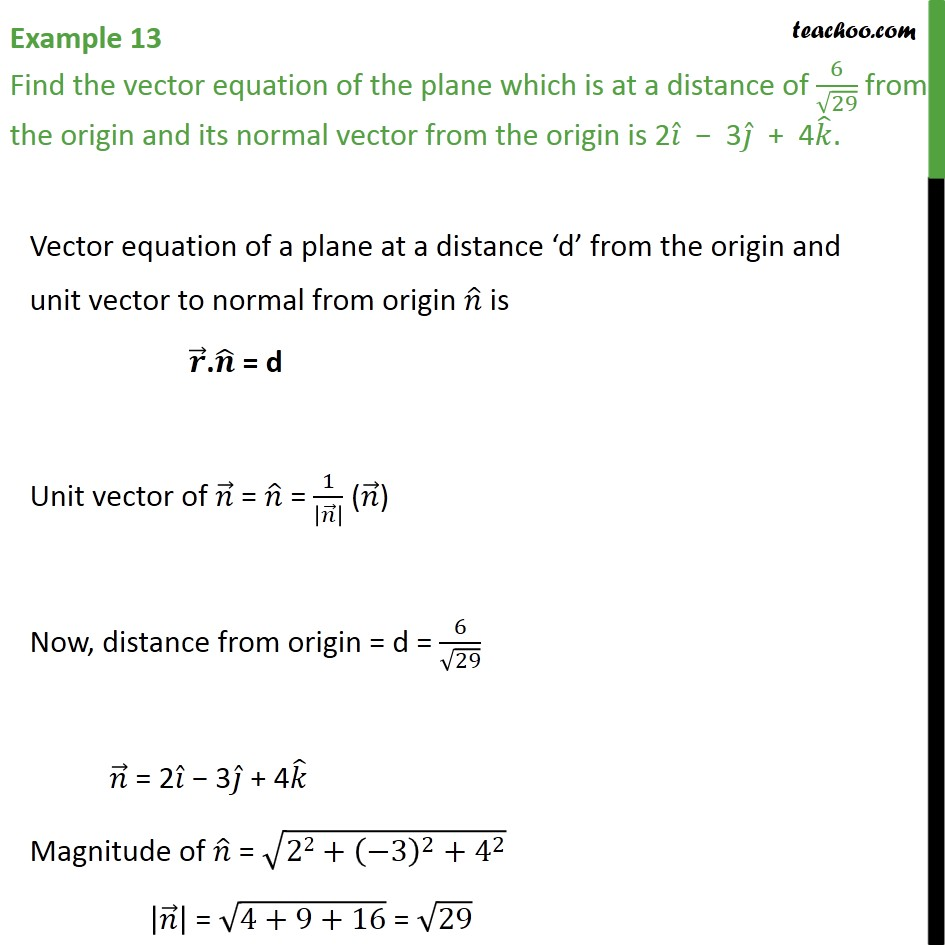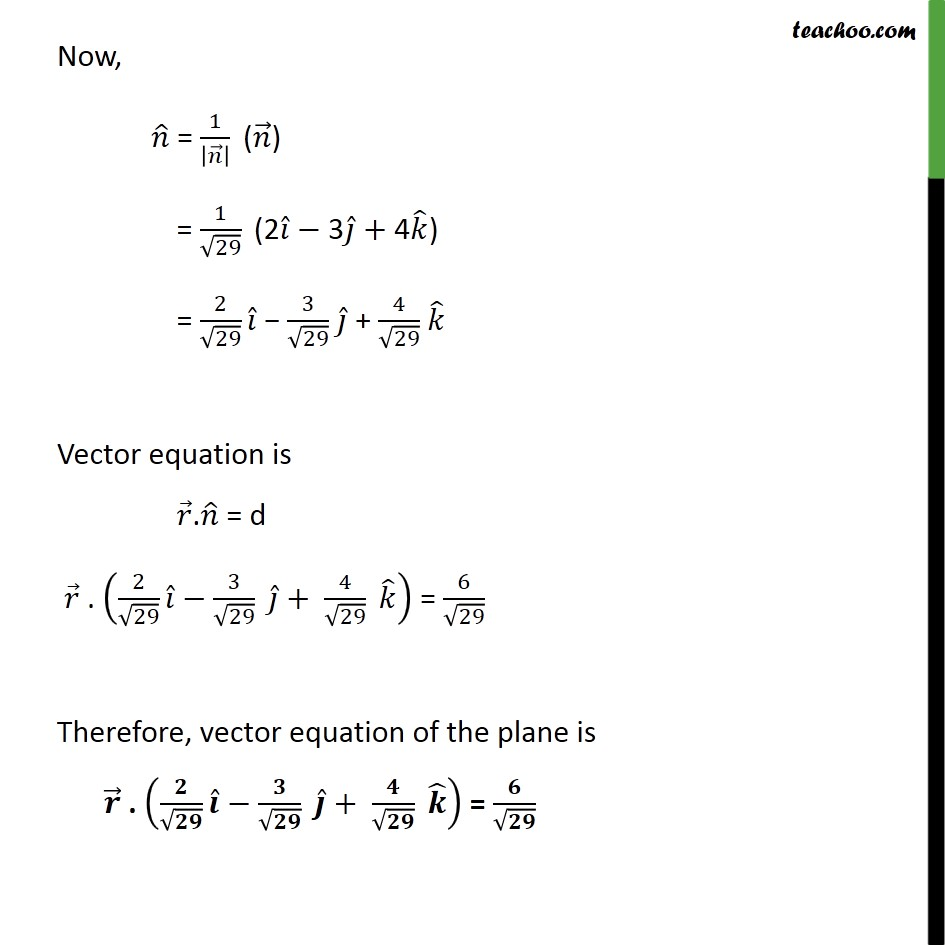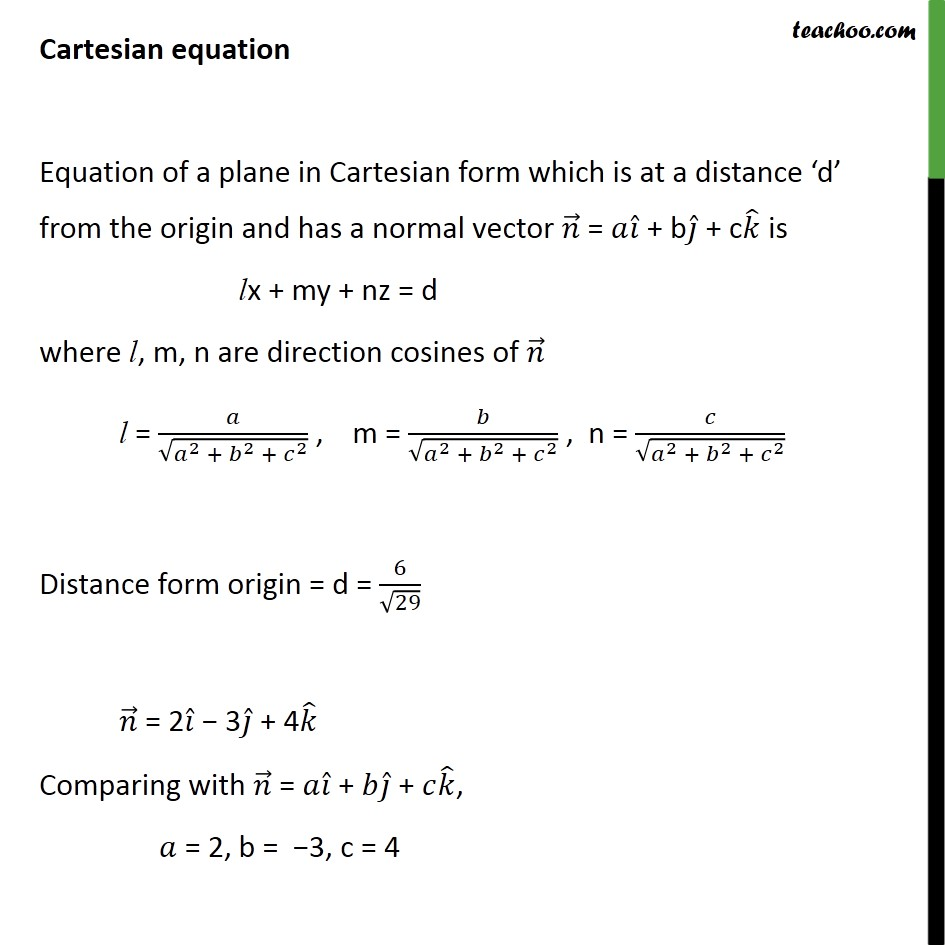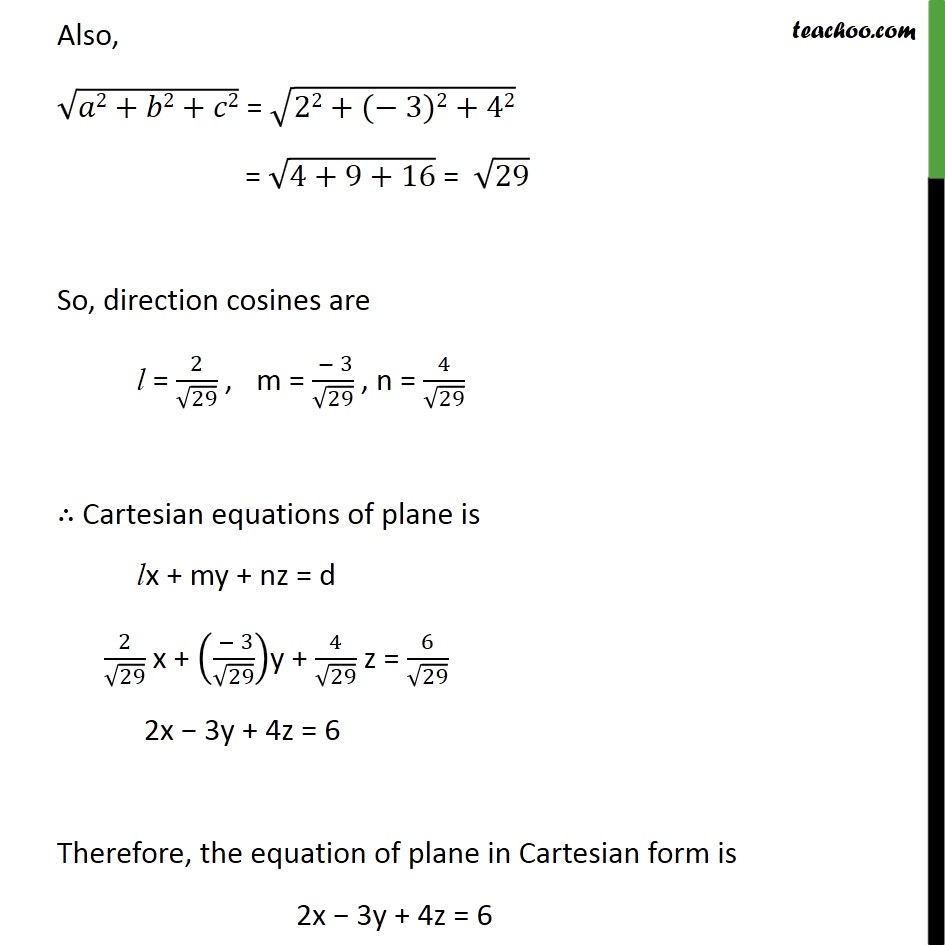1. Chapter 11 Class 12 Three Dimensional Geometry
2. Concept wise
3. Equation of plane - In Normal Form

Transcript

Example 13 Find the vector equation of the plane which is at a distance of 6 29 from the origin and its normal vector from the origin is 2 3 + 4 . Vector equation of a plane at a distance d from the origin and unit vector to normal from origin is . = d Unit vector of = = 1 ( ) Now, distance from origin = d = 6 29 = 2 3 + 4 Magnitude of = 22+ 3 2 + 4 2 = 4+9+16 = 29 Now, = 1 ( ) = 1 29 (2 3 +4 ) = 2 29 3 29 + 4 29 Vector equation is . = d . 2 29 3 29 + 4 29 = 6 29 Therefore, vector equation of the plane is . + = Cartesian equation Equation of a plane in Cartesian form which is at a distance d from the origin and has a normal vector = + b + c is lx + my + nz = d where l, m, n are direction cosines of l = 2 + 2 + 2 , m = 2 + 2 + 2 , n = 2 + 2 + 2 Distance form origin = d = 6 29 = 2 3 + 4 Comparing with = + + , = 2, b = 3, c = 4 Also, 2+ 2+ 2 = 22+( 3)2+42 = 4+9+16 = 29 So, direction cosines are l = 2 29 , m = 3 29 , n = 4 29 Cartesian equations of plane is lx + my + nz = d 2 29 x + 3 29 y + 4 29 z = 6 29 2x 3y + 4z = 6 Therefore, the equation of plane in Cartesian form is 2x 3y + 4z = 6

Equation of plane - In Normal Form

About the AuthorDavneet Singh
Davneet Singh is a graduate from Indian Institute of Technology, Kanpur. He has been teaching from the past 9 years. He provides courses for Maths and Science at Teachoo.